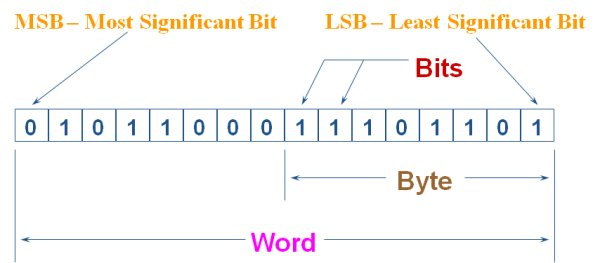#Return to the numbers pages | Decimal Numbers | Binary Numbers | Hexadecimal Numbers | Octal Numbers | BCD Number | Negative Numbers | Number Conversions

The binary number system is used by all computers, PLC/PAC's and digital device.

The binary number system is a base-2 number system, therefore there are two valid digits:

0 and 1

One digit of binary is called a bit. Bits are used in groups to represent all other numbers. Bit grouping nomiclature is as follows:

• 1 binary digit is a bit
• 4-bits is a nibble (term is not used often)
• 8-bits is a byte
• 16-bits is a word
• 32-bits is a double word or DWORD
• 64-bits is a quad word or QWORD

The binary number system is used in computers and PLC/PAC's because it is easy to use a bit to represent voltage levels within the computer or PLC/PAC. A '0' = 0Vdc or Gnd and a '1' = +Vdc.

Each bit in the binary system has a position and a weight value assigned to it. Binary is a base-2 number system, therefore the weight of each bit is 2 raised to the power of the bit position.

Binary numbers can be written in several formats:

10110012  or  1011001b
in ControlLogix: 2#1011001

The figure below depicts a 16-bit binary word showing terminology and the weight values of each bit location. Bit location zero is the bit on the far right and bit 15 is the bit on the far left.## Binary Weighting